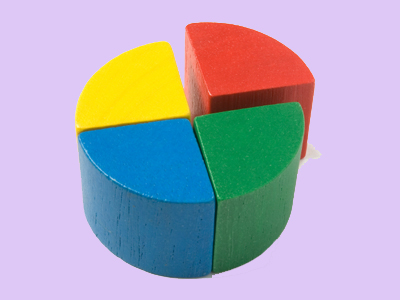If something is quartered it is divided into 4 equally sized parts.

# Year 1 Fractions - Quarters

This quiz addresses the requirements of the National Curriculum KS1 Maths and Numeracy for children aged 5 and 6 in year 1. Specifically this quiz is aimed at the section dealing with recognising, finding and naming a quarter as one of four equal parts of an object, shape or quantity. It gently introduces them to fractions.

Finding a quarter of a shape or set of objects is a progression from learning how to find a half. Children may relate finding a quarter to cutting objects into four equal parts, dividing by four or sharing groups into four equal amounts. Children should recognise that for the quarters to be accurate, all four parts or groups should be identical in size or amount.

Before you dive into the quizzes, have a read of our Fractions for Children article.

Question 1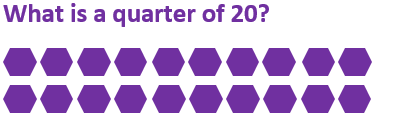24
4
15
5
Sharing 20 into four equal groups would give 5 in each group
Question 2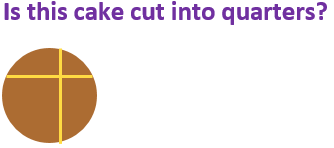Yes, there are four pieces
Yes, it has been shared between four people
Yes, there are enough pieces for four people
No, the four pieces aren’t the same size
All four quarters of a shape must be identical
Question 3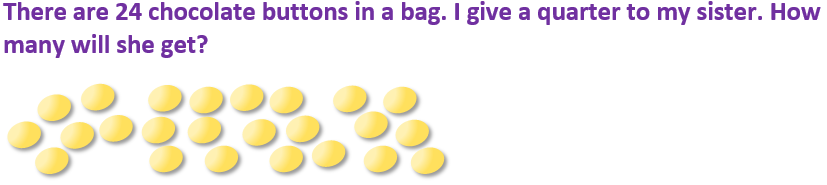6
8
4
16
24 ÷ 4 = 6, so she would get 6 buttons
Question 42
16
6
4
Using a quarter of the eggs would mean using two eggs, so 6 would be left
Question 5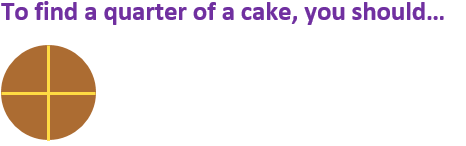Cut it into 2 slices
Cut it into 4 equal parts
Share it with some friends
Eat some and share the rest
The four quarters should be exactly the same size
Question 6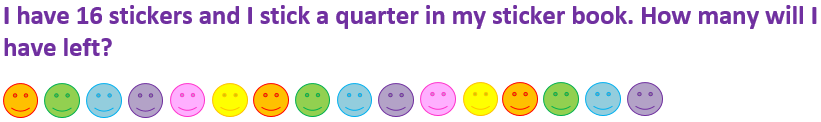16
4
8
12
A quarter of 16 is 4. 16 – 4 = 12
Question 7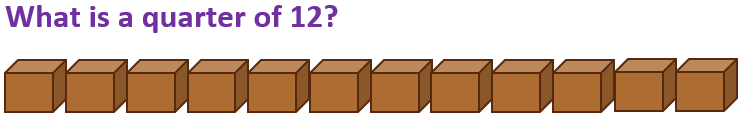3
14
20
35
12 shared into 4 equal groups gives 3 in each group, so a quarter of 12 is 3
Question 8Cut some out
Share them into 4 equal groups
Put them into 5 piles
Put them in a bag
Each group should have exactly the same number of stars
Question 9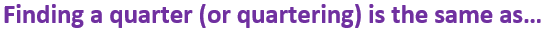Subtracting 4
Multiplying by 4
Dividing by 4
Quartering means sharing into 4 equal groups or parts
Question 10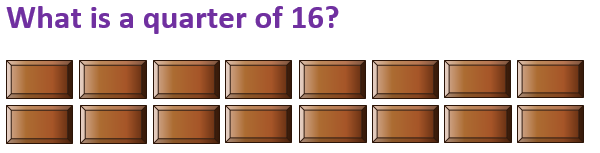4
8
16
20
16 ÷ 4 = 4, so a quarter of 16 is 4